## Insertion Sort-

• Insertion sort is an in-place sorting algorithm.
• It uses no auxiliary data structures while sorting.
• It is inspired from the way in which we sort playing cards.

## How Insertion Sort Works?

Consider the following elements are to be sorted in ascending order-

6, 2, 11, 7, 5

Insertion sort works as-

Firstly,

• It selects the second element (2).
• It checks whether it is smaller than any of the elements before it.
• Since 2 < 6, so it shifts 6 towards right and places 2 before it.
• The resulting list is 2, 6, 11, 7, 5.

Secondly,

• It selects the third element (11).
• It checks whether it is smaller than any of the elements before it.
• Since 11 > (2, 6), so no shifting takes place.
• The resulting list remains the same.

Thirdly,

• It selects the fourth element (7).
• It checks whether it is smaller than any of the elements before it.
• Since 7 < 11, so it shifts 11 towards right and places 7 before it.
• The resulting list is 2, 6, 7, 11, 5.

Fourthly,

• It selects the fifth element (5).
• It checks whether it is smaller than any of the elements before it.
• Since 5 < (6, 7, 11), so it shifts (6, 7, 11) towards right and places 5 before them.
• The resulting list is 2, 5, 6, 7, 11.

As a result, sorted elements in ascending order are-

2, 5, 6, 7, 11

## Insertion Sort Algorithm-

Let A be an array with n elements. The insertion sort algorithm used for sorting is as follows-

```for (i = 1 ; i < n ; i++)

{

key = A [ i ];

j = i - 1;

while(j > 0 && A [ j ] > key)

{

A [ j+1 ] = A [ j ];

j--;

}

A [ j+1 ] = key;

}```

Here,

• i = variable to traverse the array A
• key = variable to store the new number to be inserted into the sorted sub-array
• j = variable to traverse the sorted sub-array

## Insertion Sort Example-

Consider the following elements are to be sorted in ascending order-

6, 2, 11, 7, 5

The above insertion sort algorithm works as illustrated below-

### Step-01: For i = 1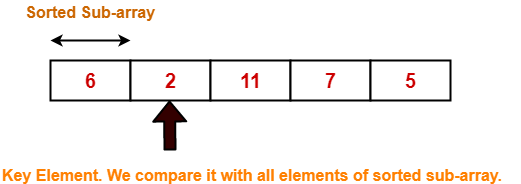### Step-02: For i = 2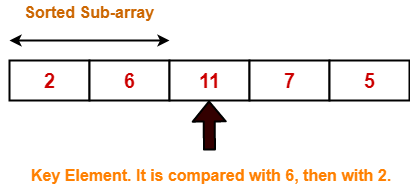### Step-03: For i = 3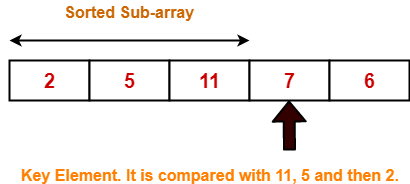2 5 11 7 6 For j = 2; 11 > 7 so A = 11 2 5 11 11 6 For j = 1; 5 < 7 so loop stops and A = 7 2 5 7 11 6 After inner loop ends

Working of inner loop when i = 3

### Step-04: For i = 4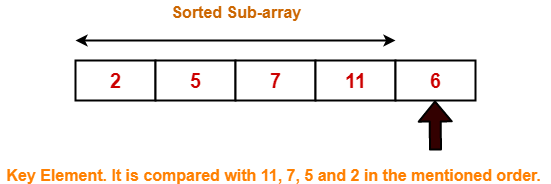Loop gets terminated as ‘i’ becomes 5. The state of array after the loops are finished-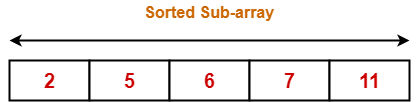With each loop cycle,

• One element is placed at the correct location in the sorted sub-array until array A is completely sorted.

## Time Complexity Analysis-

• Selection sort algorithm consists of two nested loops.
• Owing to the two nested loops, it has O(n2) time complexity.

 Time Complexity Best Case n Average Case n2 Worst Case n2

## Space Complexity Analysis-

• Selection sort is an in-place algorithm.
• It performs all computation in the original array and no other array is used.
• Hence, the space complexity works out to be O(1).

## Important Notes-

• Insertion sort is not a very efficient algorithm when data sets are large.
• This is indicated by the average and worst case complexities.
• Insertion sort is adaptive and number of comparisons are less if array is partially sorted.

To gain better understanding about Insertion Sort Algorithm,

Watch this Video Lecture

Next Article- Quick Sort

Get more notes and other study material of Design and Analysis of Algorithms.

Watch video lectures by visiting our YouTube channel LearnVidFun.

## Selection Sort-

• Selection sort is one of the easiest approaches to sorting.
• It is inspired from the way in which we sort things out in day to day life.
• It is an in-place sorting algorithm because it uses no auxiliary data structures while sorting.

## How Selection Sort Works?

Consider the following elements are to be sorted in ascending order using selection sort-

6, 2, 11, 7, 5

Selection sort works as-

• It finds the first smallest element (2).
• It swaps it with the first element of the unordered list.
• It finds the second smallest element (5).
• It swaps it with the second element of the unordered list.
• Similarly, it continues to sort the given elements.

As a result, sorted elements in ascending order are-

2, 5, 6, 7, 11

## Selection Sort Algorithm-

Let A be an array with n elements. Then, selection sort algorithm used for sorting is as follows-

```for (i = 0 ; i < n-1 ; i++)

{

index = i;

for(j = i+1 ; j < n ; j++)

{

if(A[j] < A[index])

index = j;

}

temp = A[i];

A[i] = A[index];

A[index] = temp;

}```

Here,

• i = variable to traverse the array A
• index = variable to store the index of minimum element
• j = variable to traverse the unsorted sub-array
• temp = temporary variable used for swapping

## Selection Sort Example-

Consider the following elements are to be sorted in ascending order-

6, 2, 11, 7, 5

The above selection sort algorithm works as illustrated below-

### Step-01: For i = 0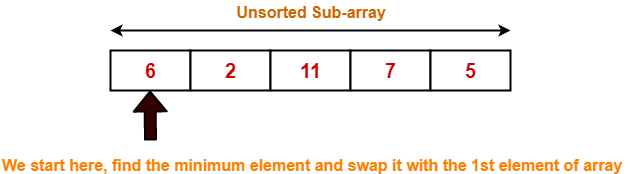### Step-02: For i = 1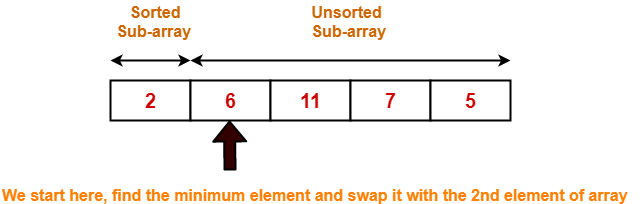### Step-03: For i = 2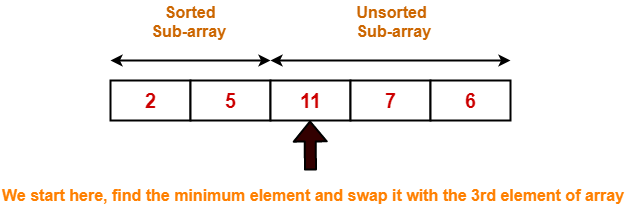### Step-04: For i = 3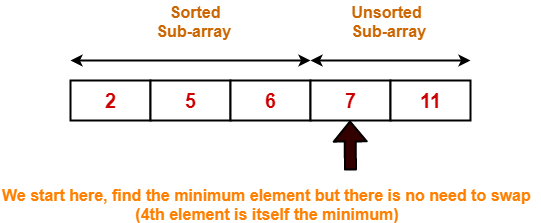### Step-05: For i = 4

Loop gets terminated as ‘i’ becomes 4.

The state of array after the loops are finished is as shown-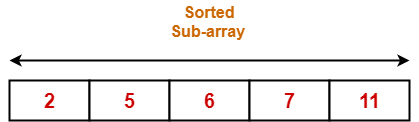With each loop cycle,

• The minimum element in unsorted sub-array is selected.
• It is then placed at the correct location in the sorted sub-array until array A is completely sorted.

## Time Complexity Analysis-

• Selection sort algorithm consists of two nested loops.
• Owing to the two nested loops, it has O(n2) time complexity.

 Time Complexity Best Case n2 Average Case n2 Worst Case n2

## Space Complexity Analysis-

• Selection sort is an in-place algorithm.
• It performs all computation in the original array and no other array is used.
• Hence, the space complexity works out to be O(1).

## Important Notes-

• Selection sort is not a very efficient algorithm when data sets are large.
• This is indicated by the average and worst case complexities.
• Selection sort uses minimum number of swap operations O(n) among all the sorting algorithms.

To gain better understanding about Selection Sort Algorithm,

Watch this Video Lecture

Next Article- Insertion Sort

Get more notes and other study material of Design and Analysis of Algorithms.

Watch video lectures by visiting our YouTube channel LearnVidFun.

## Prim’s and Kruskal’s Algorithms-

Before you go through this article, make sure that you have gone through the previous articles on Prim’s Algorithm & Kruskal’s Algorithm.

We have discussed-

• Prim’s and Kruskal’s Algorithm are the famous greedy algorithms.
• They are used for finding the Minimum Spanning Tree (MST) of a given graph.
• To apply these algorithms, the given graph must be weighted, connected and undirected.

Some important concepts based on them are-

## Concept-01:

If all the edge weights are distinct, then both the algorithms are guaranteed to find the same MST.

### Example-

Consider the following example-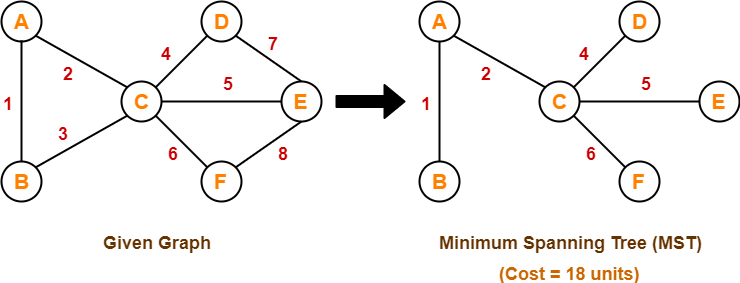Here, both the algorithms on the above given graph produces the same MST as shown.

## Concept-02:

• If all the edge weights are not distinct, then both the algorithms may not always produce the same MST.
• However, cost of both the MSTs would always be same in both the cases.

### Example-

Consider the following example-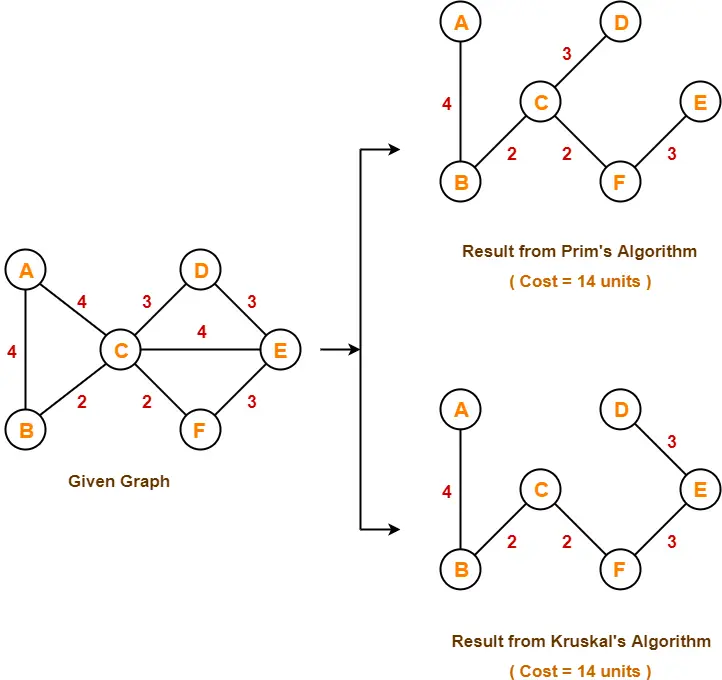Here, both the algorithms on the above given graph produces different MSTs as shown but the cost is same in both the cases.

## Concept-03:

Kruskal’s Algorithm is preferred when-

• The graph is sparse.
• There are less number of edges in the graph like E = O(V)
• The edges are already sorted or can be sorted in linear time.

Prim’s Algorithm is preferred when-

• The graph is dense.
• There are large number of edges in the graph like E = O(V2).

## Concept-04:

Difference between Prim’s Algorithm and Kruskal’s Algorithm-

 Prim’s Algorithm Kruskal’s Algorithm The tree that we are making or growing always remains connected. The tree that we are making or growing usually remains disconnected. Prim’s Algorithm grows a solution from a random vertex by adding the next cheapest vertex to the existing tree. Kruskal’s Algorithm grows a solution from the cheapest edge by adding the next cheapest edge to the existing tree / forest. Prim’s Algorithm is faster for dense graphs. Kruskal’s Algorithm is faster for sparse graphs.

To gain better understanding about Difference between Prim’s and Kruskal’s Algorithm,

Watch this Video Lecture

Next Article- Linear Search

Get more notes and other study material of Design and Analysis of Algorithms.

Watch video lectures by visiting our YouTube channel LearnVidFun.

## Kruskal’s Algorithm-

• Kruskal’s Algorithm is a famous greedy algorithm.
• It is used for finding the Minimum Spanning Tree (MST) of a given graph.
• To apply Kruskal’s algorithm, the given graph must be weighted, connected and undirected.

## Kruskal’s Algorithm Implementation-

The implementation of Kruskal’s Algorithm is explained in the following steps-

### Step-01:

• Sort all the edges from low weight to high weight.

### Step-02:

• Take the edge with the lowest weight and use it to connect the vertices of graph.
• If adding an edge creates a cycle, then reject that edge and go for the next least weight edge.

### Step-03:

• Keep adding edges until all the vertices are connected and a Minimum Spanning Tree (MST) is obtained.

### Thumb Rule to Remember

The above steps may be reduced to the following thumb rule-

• Simply draw all the vertices on the paper.
• Connect these vertices using edges with minimum weights such that no cycle gets formed.

## Kruskal’s Algorithm Time Complexity-

 Worst case time complexity of Kruskal’s Algorithm= O(ElogV) or O(ElogE)

### Analysis-

• The edges are maintained as min heap.
• The next edge can be obtained in O(logE) time if graph has E edges.
• Reconstruction of heap takes O(E) time.
• So, Kruskal’s Algorithm takes O(ElogE) time.
• The value of E can be at most O(V2).
• So, O(logV) and O(logE) are same.

### Special Case-

• If the edges are already sorted, then there is no need to construct min heap.
• So, deletion from min heap time is saved.
• In this case, time complexity of Kruskal’s Algorithm = O(E + V)

## Problem-01:

Construct the minimum spanning tree (MST) for the given graph using Kruskal’s Algorithm-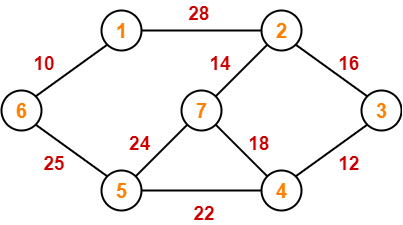## Solution-

To construct MST using Kruskal’s Algorithm,

• Simply draw all the vertices on the paper.
• Connect these vertices using edges with minimum weights such that no cycle gets formed.

### Step-01: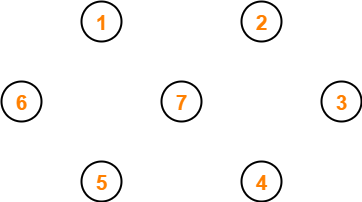### Step-02: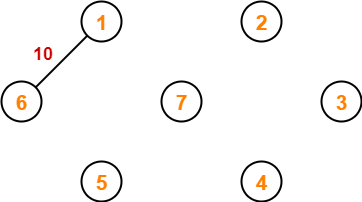### Step-03: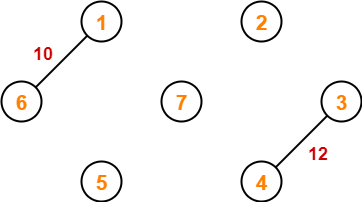### Step-04: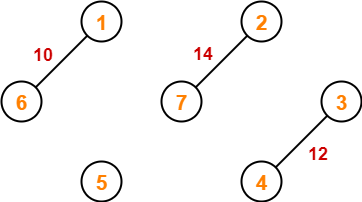### Step-05: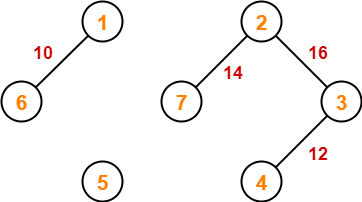### Step-06: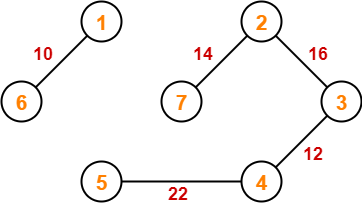### Step-07: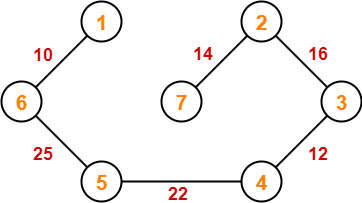Since all the vertices have been connected / included in the MST, so we stop.

Weight of the  MST

= Sum of all edge weights

= 10 + 25 + 22 + 12 + 16 + 14

= 99 units

To gain better understanding about Kruskal’s Algorithm,

Watch this Video Lecture

To practice previous years GATE problems based on Kruskal’s Algorithm,

Watch this Video Lecture

Next Article- Prim’s Algorithm Vs Kruskal’s Algorithm

Get more notes and other study material of Design and Analysis of Algorithms.

Watch video lectures by visiting our YouTube channel LearnVidFun.

## Prim’s Algorithm-

• Prim’s Algorithm is a famous greedy algorithm.
• It is used for finding the Minimum Spanning Tree (MST) of a given graph.
• To apply Prim’s algorithm, the given graph must be weighted, connected and undirected.

## Prim’s Algorithm Implementation-

The implementation of Prim’s Algorithm is explained in the following steps-

### Step-01:

• Randomly choose any vertex.
• The vertex connecting to the edge having least weight is usually selected.

### Step-02:

• Find all the edges that connect the tree to new vertices.
• Find the least weight edge among those edges and include it in the existing tree.
• If including that edge creates a cycle, then reject that edge and look for the next least weight edge.

### Step-03:

• Keep repeating step-02 until all the vertices are included and Minimum Spanning Tree (MST) is obtained.

## Prim’s Algorithm Time Complexity-

Worst case time complexity of Prim’s Algorithm is-

• O(ElogV) using binary heap
• O(E + VlogV) using Fibonacci heap

### Time Complexity Analysis

• If adjacency list is used to represent the graph, then using breadth first search, all the vertices can be traversed in O(V + E) time.
• We traverse all the vertices of graph using breadth first search and use a min heap for storing the vertices not yet included in the MST.
• To get the minimum weight edge, we use min heap as a priority queue.
• Min heap operations like extracting minimum element and decreasing key value takes O(logV) time.

So, overall time complexity

= O(E + V) x O(logV)

= O((E + V)logV)

= O(ElogV)

This time complexity can be improved and reduced to O(E + VlogV) using Fibonacci heap.

## Problem-01:

Construct the minimum spanning tree (MST) for the given graph using Prim’s Algorithm-## Solution-

The above discussed steps are followed to find the minimum cost spanning tree using Prim’s Algorithm-

### Step-01: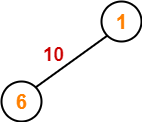### Step-02: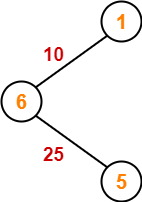### Step-03: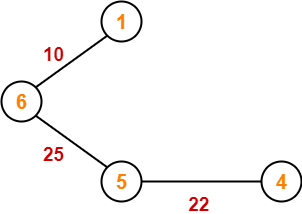### Step-04: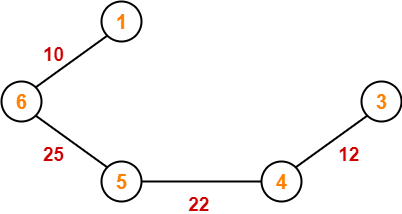### Step-05: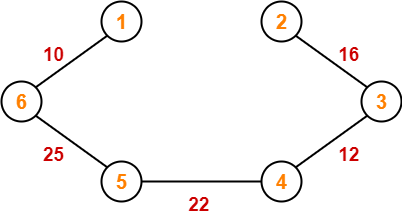### Step-06: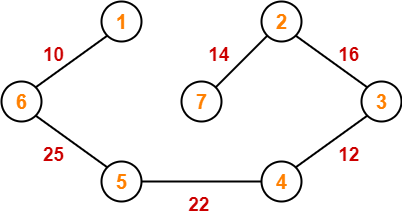Since all the vertices have been included in the MST, so we stop.

Now, Cost of Minimum Spanning Tree

= Sum of all edge weights

= 10 + 25 + 22 + 12 + 16 + 14

= 99 units

## Problem-02:

Using Prim’s Algorithm, find the cost of minimum spanning tree (MST) of the given graph-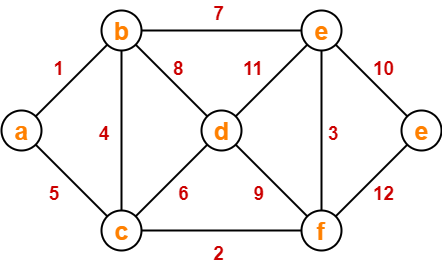## Solution-

The minimum spanning tree obtained by the application of Prim’s Algorithm on the given graph is as shown below-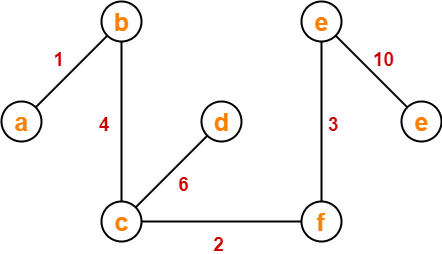Now, Cost of Minimum Spanning Tree

= Sum of all edge weights

= 1 + 4 + 2 + 6 + 3 + 10

= 26 units

To gain better understanding about Prim’s Algorithm,

Watch this Video Lecture

To practice previous years GATE problems based on Prim’s Algorithm,

Watch this Video Lecture

Next Article- Kruskal’s Algorithm

Get more notes and other study material of Design and Analysis of Algorithms.

Watch video lectures by visiting our YouTube channel LearnVidFun.